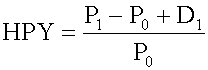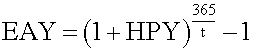### Why should I choose AnalystNotes?

Simply put: AnalystNotes offers the best value and the best product available to help you pass your exams.

### Subject 4. Different Yield Measures of a U.S. Treasury Bill

Money market instruments are low-risk, highly liquid debt instruments with a maturity of one year or less. There are two types of money market instruments: interest-bearing instruments (e.g., bank certificates of deposit), and pure discount instruments (e.g., U.S. Treasury bills).

Pure discount instruments such as T-bills are quoted differently than U.S. government bonds. They are quoted on a bank discount basis rather than on a price basis:• rBD = the annualized yield on a bank discount basis
• D = the dollar discount, which is equal to the difference between the face value of the bill, F, and its purchase price, P
• t = the number of days remaining to maturity
• 360 = the bank convention of the number of days in a year.

Bank discount yield is not a meaningful measure of the return on investment because:

• It is based on the face value, not on the purchase price. Instead, return on investment should be measured based on cost of investment.
• It is annualized using a 360-day year, not a 365-day year.
• It annualizes with simple interest and ignores the effect of interest on interest (compound interest).

Holding period yield (HPY) is the return earned by an investor if the money market instrument is held until maturity:• P0 = the initial price of the instrument
• P1 = the price received for the instrument at its maturity
• D1 = the cash distribution paid by the instrument at its maturity (that is, interest).

Since a pure discount instrument (e.g., a T-bill) makes no interest payment, its HPY is (P1 - P0)/P0.

Note that HPY is computed on the basis of purchase price, not face value. It is not an annualized yield.

The effective annual yield is the annualized HPY on the basis of a 365-day year. It incorporates the effect of compounding interest.Money market yield (also known as CD equivalent yield) is the annualized HPY on the basis of a 360-day year using simple interest.Example

An investor buys a \$1,000 face-value T-bill due in 60 days at a price of \$990.

• Bank discount yield: (1000 - 990)/1000 x 360/60 = 6%
• Holding period yield: (1000 - 990)/990 = 1.0101%
• Effective annual yield: (1 + 1.0101%)365/60 - 1 = 6.3047%
• Money market yield: (360 x 6%)/(360 - 60 x 6%) = 6.0606%

If we know HPY, then:

• EAY = (1 + HPY)365/t - 1
• rMM = HPY x 360/t

If we know EAY, then:

• HPY = ( 1 + EAY)t/365 - 1
• rMM = [(1 + EAY)t/365 - 1] x (360/t)

If we know rMM, then:

• HPY = rMM x (t/360)
• EAY = (1 + rMM x t/360)365/t - 1

#### Practice Question 1

The price of a six-month (182-day) U.S. Treasury bill with a par value of \$100,000 and a bank discount yield of 9.18 percent is ______.

A. \$90,720
B. \$95,359
C. \$97,680

#### Practice Question 2

A Treasury bill with 50 days till maturity is quoted with a bank discount rate of 3.50%. An investor purchasing \$2,000,000 face value of this Treasury bill would pay ______.

A. \$1,965,221.84
B. \$1,930,000.00
C. \$1,990,277.78

F = \$2,000,000
D = 0.035*(50/360)*\$2,000,000 = \$9,722.22
P = \$2,000,000 - \$9,722.22 = \$1,990,277.78

#### Practice Question 3

Given a 30-day horizon, a money market yield of 4.0134% produces a holding period yield of ______.

A. 0.33445%
B. 0.4213%
C. 0.3455%

MMY -> HPY
HPY = 0.040134 /(360/30) = 0.33445%

#### Practice Question 4

On June 1, 2008, the 12 3/8 May '14 Treasury bond is quoted at 134:05 bid and 134:09 asked. If Smedley buys a bond at the market rate and if the value of the bond on December 1, 2008 is 132:00, what will be her holding period return? (Do not take accrued interest, if any, into account in calculating the return)

A. 1.0300
B. 2.91%
C. 1.0291

HPR = (Price1 + Interest - Price0) /Price0
One interest payment of 6.1875 will be received in November. This is 12.375/2.
Buying at the market, Smedley will pay the asked price of 134 9/32 or 134.28125.
HPR = (132 + 6.1875 - 134.28125)/134.28125 = 0.0291

#### Practice Question 5

A Treasury bill with 50 days till maturity is quoted with a bank discount rate of 3.50%. The holding period yield for this T-bill, if purchased and held to maturity, would be closest to ______.

A. .4861%
B. .4885%
C. 3.50%

Holding period yield calculated:
F = \$1,000
D = 0.035*(50/360)*\$1,000 = \$4.8611
P = \$1,000 - \$4.8611 = \$995.1389
HPY = \$4.8611/\$995.1389 = 0.4885%

#### Practice Question 6

A 30-day T-bill is selling at a money market yield of 2.95%. What is its equivalent bank discount yield?

A. 2.94%
B. 2.95%
C. 2.97%

Based on money market yield:

0.0295 = [(100,000 - P)/P] x (360/30) => P = 100,000/[1+0.0295/12] = 99,754.8
Bank discount yield = [(100,000 - 99,754.8)/100,000] x (360/30) = 0.0294, or 2.94%.

#### Practice Question 7

A 180-day U.S. Treasury bill has a holding period yield (HPY) of 2.375%. The bank discount yield (in %) is closest to ______.

A. 4.640
B. 4.780
C. 7.850